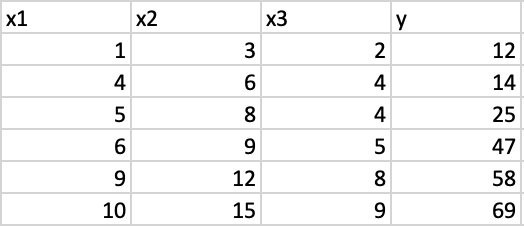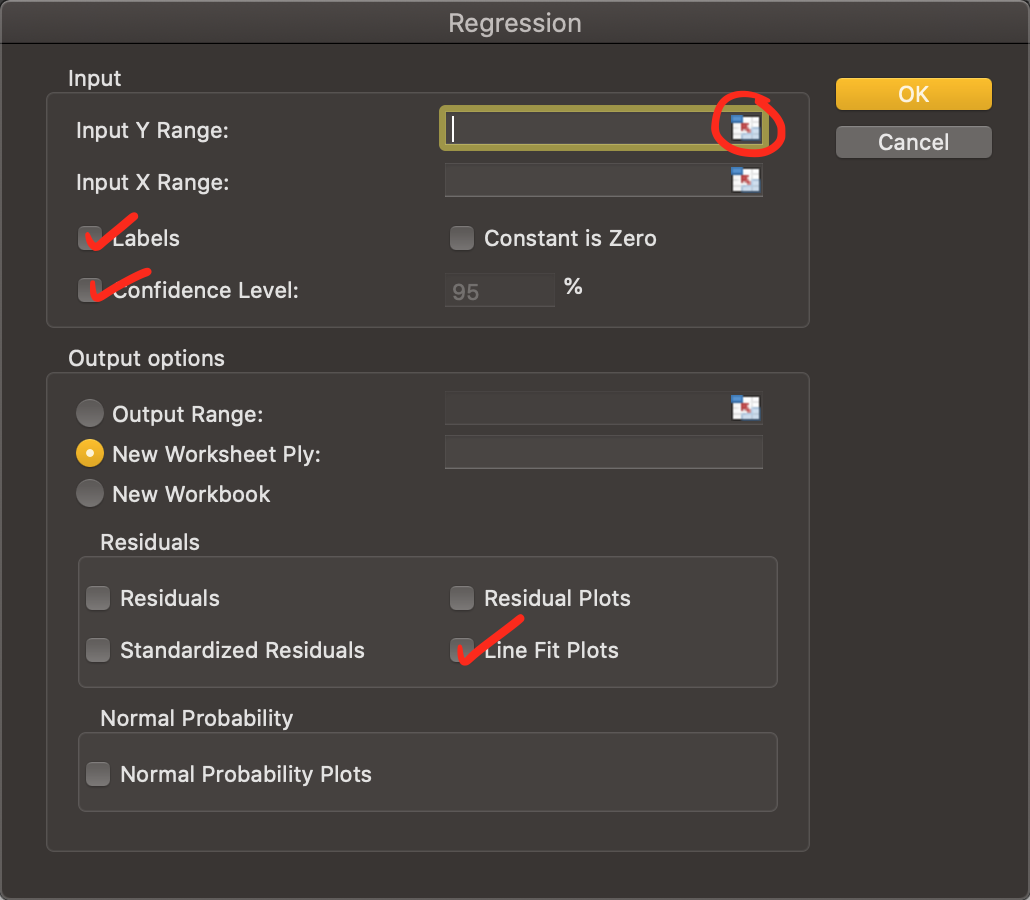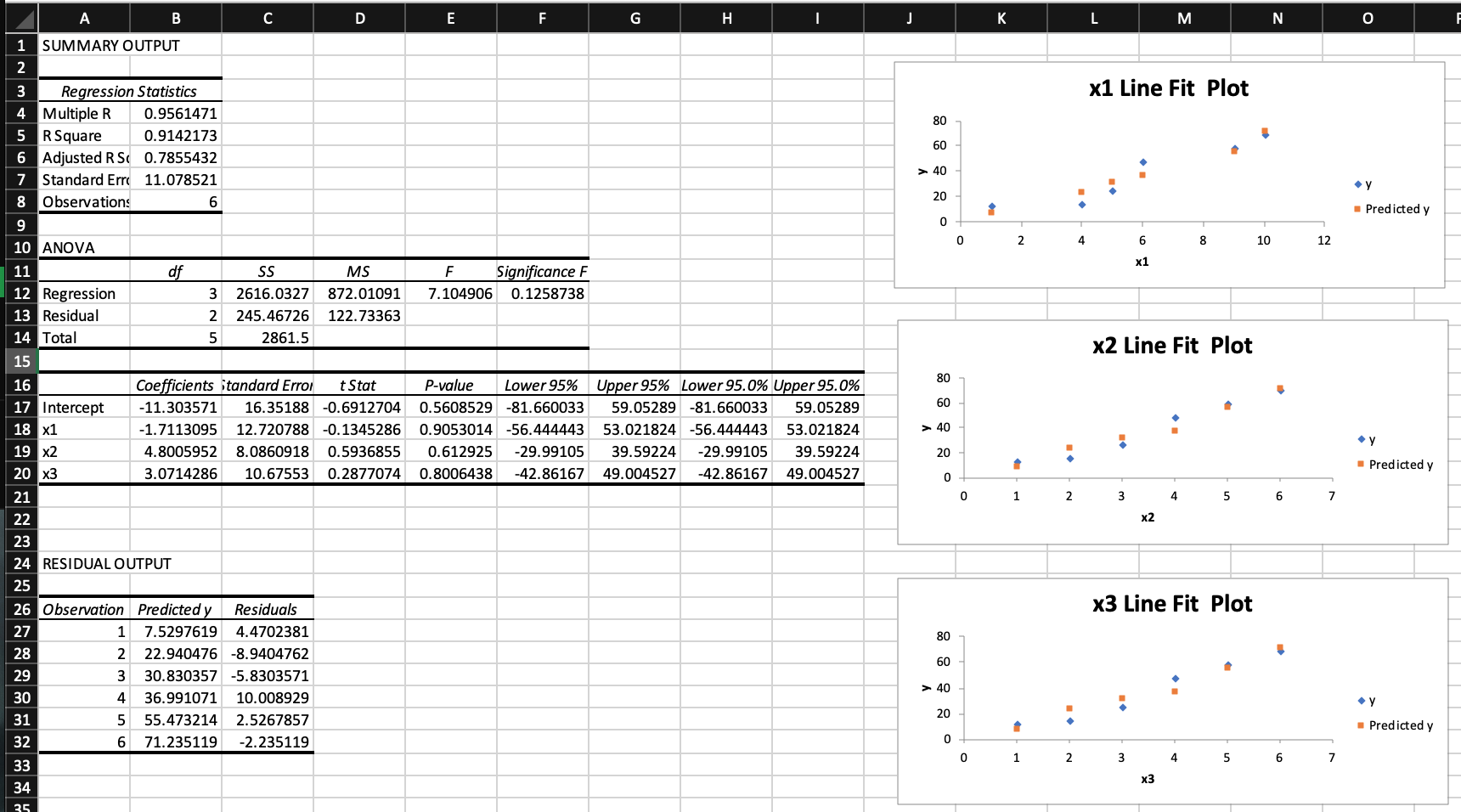1597335660

# Ways to Evaluate Regression Models

The very naive way of evaluating a model is by considering the R-Squared value. Suppose if I get an R-Squared of 95%, is that good enough? Through this blog, Let us try and understand the ways to evaluate your regression model.

### Evaluation metrics;

• Mean/Median of prediction
• Standard Deviation of prediction
• Range of prediction
• Coefficient of Determination (R2)
• Relative Standard Deviation/Coefficient of Variation (RSD)
• Relative Squared Error (RSE)
• Mean Absolute Error (MAE)
• Relative Absolute Error (RAE)
• Mean Squared Error (MSE)
• Root Mean Squared Error on Prediction (RMSE/RMSEP)
• Normalized Root Mean Squared Error (Norm RMSEP)
• Relative Root Mean Squared Error (RRMSEP)

#rmse #machine-learning #regression #data-science #metrics

## Buddha Community1594017960

# Introduction

Excel is a powerful data manipulation and analysis tool that, once mastered, can entirely replace the lengthy and confusing coding that comes with data analysis in Python, R, and the like. This article is an example of one of those Excel tools: creating single and multiple linear regression models that output a plethora of neatly arranged statistics, graphs, and insights, with the click of just a few buttons.

Regressions are used to model the relationship between one or more independent variables and their corresponding dependent variable. This is done by fitting a line (“line of best fit”) between the variables, which can aid in predicting future outcomes — regressions are a handy data science tool, made even simpler with data analysis in Excel.

# Tutorial

Begin by storing all independent/x-values in one column (if using multiple independent variables, store each in its own column), and the dependent/y-value in a separate column. The small table should look something like this:Next, head to the “Data” tab, and click on the “Data Analysis” button in the upper right corner of the screen. Don’t see a Data Analysis button?

1. At the top of your screen, select “Tools”, and click on“Excel Add-ins”.
2. Check the box next to “Analysis ToolPak” and click OK.

The Data Analysis button should appear immediately. After having clicked on the button, scroll and select “Regression” from the list (and click OK).Now, there are several different boxes and inputs in the pop-up on the screen, but don’t be alarmed! The process for inputting data is easy. Begin by clicking on the mini-sheet icon in the “Input Y Range” box, and select the ENTIRE column of data, INCLUDING the column name. Click enter, and the data range appears in the input box. Repeat the same process for the “Input X Range” box, with either one single or multiple columns selected. Check the boxes selected in the above screenshot, and hit OK.

And that’s it! The regression should pop up in a new sheet, unless otherwise specified — and with a LOT of statistical data.The most important numbers here are included in the “Regression Statistics” box: Multiple R and R Squared return the correlation strength/accuracy of the regression, and are important to evaluating if the linear regression is a good model. The graphs are helpful in visualizing the same concept, and are great ways to explain the regression to a non-technical audience.

# Conclusion

As demonstrated above, creating linear regressions in Excel is VERY easy. All of the returned statistics, tests, and graphs would have required endless manual coding on other platforms, but were generated in under 5 minutes using Excel’s Data Analysis tool. I hope this article helps speed up your data analysis, and does the capabilities of Excel some justice!

#data-science #regression #excel #data-analysis #modeling #data analysis1598352300

## Regression: Linear Regression

Machine learning algorithms are not your regular algorithms that we may be used to because they are often described by a combination of some complex statistics and mathematics. Since it is very important to understand the background of any algorithm you want to implement, this could pose a challenge to people with a non-mathematical background as the maths can sap your motivation by slowing you down.In this article, we would be discussing linear and logistic regression and some regression techniques assuming we all have heard or even learnt about the Linear model in Mathematics class at high school. Hopefully, at the end of the article, the concept would be clearer.

**Regression Analysis **is a statistical process for estimating the relationships between the dependent variables (say Y) and one or more independent variables or predictors (X). It explains the changes in the dependent variables with respect to changes in select predictors. Some major uses for regression analysis are in determining the strength of predictors, forecasting an effect, and trend forecasting. It finds the significant relationship between variables and the impact of predictors on dependent variables. In regression, we fit a curve/line (regression/best fit line) to the data points, such that the differences between the distances of data points from the curve/line are minimized.

#regression #machine-learning #beginner #logistic-regression #linear-regression #deep learning1592023980

## 5 Regression algorithms: Explanation & Implementation in Python

Take your current understanding and skills on machine learning algorithms to the next level with this article. What is regression analysis in simple words? How is it applied in practice for real-world problems? And what is the possible snippet of codes in Python you can use for implementation regression algorithms for various objectives? Let’s forget about boring learning stuff and talk about science and the way it works.

#linear-regression-python #linear-regression #multivariate-regression #regression #python-programming1601431200

## Polynomial Regression — The “curves” of a linear model

The most glamorous part of a data analytics project/report is, as many would agree, the one where the Machine Learning algorithms do their magic using the data. However, one of the most overlooked part of the process is the preprocessing of data.

A lot more significant effort is put into preparing the data to fit a model on rather than tuning the model to fit the data better. One such preprocessing technique that we intend to disentangle is Polynomial Regression.

#data-science #machine-learning #polynomial-regression #regression #linear-regression

1597335660

## Ways to Evaluate Regression Models

The very naive way of evaluating a model is by considering the R-Squared value. Suppose if I get an R-Squared of 95%, is that good enough? Through this blog, Let us try and understand the ways to evaluate your regression model.

### Evaluation metrics;

• Mean/Median of prediction
• Standard Deviation of prediction
• Range of prediction
• Coefficient of Determination (R2)
• Relative Standard Deviation/Coefficient of Variation (RSD)
• Relative Squared Error (RSE)
• Mean Absolute Error (MAE)
• Relative Absolute Error (RAE)
• Mean Squared Error (MSE)
• Root Mean Squared Error on Prediction (RMSE/RMSEP)
• Normalized Root Mean Squared Error (Norm RMSEP)
• Relative Root Mean Squared Error (RRMSEP)

#rmse #machine-learning #regression #data-science #metrics